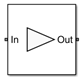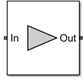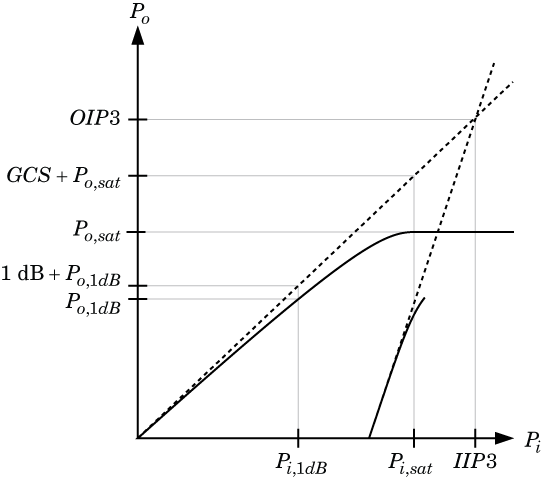# Amplifier

Model amplifier in RF systems

•Libraries:
RF Blockset / Circuit Envelope / Elements

## Description

Use the Amplifier block to model a linear or nonlinear amplifier, with or without noise. Defining the amplifier gain using a data source also defines input data visualization and modeling. Use the Main tab parameters to specify amplifier gain and noise using data sheet values, standard `s2p` files, S-parameters, or circuit envelope polynomial coefficients.

The amplifier is implemented as a polynomial, voltage-controlled voltage source (VCVS) except when the amplifier gain is obtained from a `Data source`. The VCVS includes nonlinearities that are described using parameters listed in the Nonlinearity tab. To model linear amplification, the amplifier implements the relation Vout = a1*Vin between the input and output voltages. The input voltage is Vi(t) = Ai(t)ejωt, and the output voltage is Vo(t) = Ao(t)ejωt at each carrier w = 2πf in the RF Blockset™ environment.

In case the amplifier gain is obtained from a data source, amplifier implementation is based on S-parameter data. Nonlinear amplification is modeled as a polynomial (with the saturation power computed automatically). It also produces additional intermodulation frequencies.

Amplifier block mask icons are dynamic and show the current state of the applied noise parameter. This table shows you how the icons on this block vary based on the state of the Noise figure (dB) parameter on the block.

Noise Figure (dB): `10`Noise Figure (dB): `0`## Parameters

expand all

### Main

Source of the amplifier gain, specified as one of the following:

• `Available power gain` — The block uses the Available power gain parameter to calculate a1, the linear voltage gain term of the polynomial VCVS. This calculation assumes a matched load termination for the amplifier.

• `Open circuit voltage gain` — The block uses the Open circuit voltage gain parameter to calculate a1, the linear voltage gain term of the polynomial VCVS.

• `Data source` — The block uses the Data source parameter to calculate the linear voltage gain. When you use the data source option, the block uses S11 and S22 as the input and output impedances.

• `Polynomial coefficients` — The block uses the Polynomial coefficients parameter to calculate the nonlinear voltage gain.

• `AM/AM-AM/PM table` — The block uses the AM/AM-AM/PM table parameter to determine the power characteristics of the amplifier.

Available power gain of amplifier, specified as a scalar in dB. Specify the units from the corresponding drop-down list.

#### Dependencies

To enable this parameter, choose ```Available power gain``` in the Source of amplifier gain tab.

Open circuit voltage of amplifier, specified as a scalar in dB. Specify the units from the corresponding drop-down list.

#### Dependencies

To enable this parameter, choose ```Open circuit voltage gain``` in the Source of amplifier gain tab.

Data source, specified as one of the following:

• `Data file` — Name of a Touchstone file with the extension`.s2p`.

• `Network-parameters` — Provide Network parameter data such as `S-parameters`, `Y-parameters`, and `Z-parameters` with corresponding Frequency and Reference impedance (ohms) for the amplifier.

• `Rational model` — Provide values for Residues, Poles, and Direct feedthrough parameters which correspond to the equation for a rational model

`$F\left(s\right)=\left(\sum _{k=1}^{n}\frac{{C}_{k}}{s-{A}_{k}}+D\right)\begin{array}{cc},& s=j2\pi f\end{array}$`

In this rational model equation, each Ck is the residue of the pole Ak. If Ck is complex, a corresponding complex conjugate pole and residue must also be enumerated. This object has the properties `C`, `A`, and `D`. You can use these properties to specify the Residues, Poles, and Direct feedthrough parameters.

When the amplifier is nonlinear, the nonlinearity applies only to the S21 term of the scattering parameters representing the 2-port element. In this case, S21 is frequency-independent with its constant value being either the maximal value of S21, or the S21 value at an Operation frequency specified by the user. The other scattering parameters, S11, S12, and S22 remain the same as in the linear case.

#### Dependencies

To enable this parameter, select ```Data source``` in Source of amplifier gain tab.

Order of polynomial, specified as a vector.

The order of the polynomial must be less than or equal to 9. The coefficients are ordered in ascending powers. If a vector has 10 coefficients, ```[a0,a1,a2, ... a9]```, the polynomial it represents is:

Vout = a0 + a1Vin + a2Vin2 + ...  + a9Vin9

where a1 represents the linear gain term, and higher-order terms are modeled according to .

For example, the vector `[a0,a1,a2,a3]` specifies the relation Vout = a0 + a1V1 + a2V12 + a3V13. Trailing zeroes are omitted. So, `[a0,a1,a2]` defines the same polynomial as ```[a0,a1,a2, 0]```. The default value of this parameter is [0,1], corresponding to the linear relation Vout = Vin.

#### Dependencies

To enable this parameter, select ```Polynomial coefficients``` in Source of amplifier gain tab.

Table lookup entries specified as a real M-by-3 matrix. The second column of the matrix represents the AM/AM–AM/PM model output power in dBm. The third column represents the model phase change in degrees. The second and third column values are related to the absolute value of the power of the input signal represented in the first column of matrix. The input power in the first column must increase monotonically.

#### Dependencies

To enable this parameter, set Source of amplifier gain to ```AM/AM - AM/PM table``` .

Select this parameter to use a network parameter object as the source of amplifier gain.

Once you select this parameter, in the text box that opens, specify one of these:

• Network parameter object.

• MATLAB® base workspace variable or a Simulink® model workspace variable. The block evaluates if the specified variable is a network parameter object.

The network parameter objects that you can specify include:

#### Dependencies

To enable this parameter, first select ```Data source``` in Source of amplifier gain, and then select `Network-parameters` in Data source.

Network parameter type, specified as `S-parameters`, `Y-parameters`, or `Z-parameters`.

#### Dependencies

To enable this parameter, first select ```Data source``` in Source of amplifier gain and then, select `Network-parameters` in the Data source.

Input impedance of amplifier, specified as a scalar.

#### Dependencies

To enable this parameter, select ```Available power gain```, ```Open circuit voltage gain```, or ```Polynomial coefficients``` in Source of amplifier gain.

Output impedance of amplifier, specified as a scalar in ohms.

#### Dependencies

To enable this parameter, select ```Available power gain```, ```Open circuit voltage gain```, or ```Polynomial coefficients``` in Source of amplifier gain.

Name of network parameter data file, specified as a character vector.

#### Dependencies

To enable this parameter, first select ```Data source``` in Source of amplifier gain and then, select ```Data file``` in Data source.

Frequency of network parameters, specified as a scalar in Hz.

#### Dependencies

To enable this parameter, first select ```Data source``` in Source of amplifier gain and then, select `Network-parameters` in Data source.

Reference impedance of network parameters, specified as a scalar in ohms.

#### Dependencies

To enable this parameter, first select ```Data source``` in Source of amplifier gain and then, select `Network-parameters` in Data source.

Select this parameter to use a `rational` object as the data source to model amplifier gain.

Once you select this parameter, in the text box that opens, specify one of these:

• `rational` object.

• MATLAB base workspace variable or a Simulink model workspace variable. The block evaluates if the specified variable is a rational object."

The `rational` objects that you can specify include:

#### Dependencies

To enable this parameter, first select ```Data source``` in Source of amplifier gain, and then select ```Rational model``` in Data source.

Residues in order of rational model, specified as a vector.

#### Dependencies

To enable this parameter, first select ```Data source``` in Source of amplifier gain and then, select ```Rational model``` in Data source.

Poles in order of rational model, specified as a vector.

#### Dependencies

To enable this parameter, first select ```Data source``` in Source of amplifier gain and then, select ```Rational model``` in Data source.

Direct feedthrough, specified as an array vector.

#### Dependencies

To enable this parameter, first select ```Data source``` in Source of amplifier gain and then, select ```Rational model``` in Data source.

Select this option to ground and hide the negative terminals. Clear this parameter to expose the negative terminals. By exposing these terminals, you can connect them to other parts of your model.

By default, this option is selected.

### Nonlinearity

Type of nonlinearity, specified as ```Even and odd order``` or `Odd order`.

• When you select `Even and odd order`, the amplifier can produce second- and third-order intermodulation frequencies in addition to a linear term.

• When you select `Odd order`, the amplifier generates only odd order intermodulation frequencies.

The linear gain determines the linear a1 term. The block calculates the remaining terms from the specified parameters. These parameters are IP3, 1-dB gain compression power, Output saturation power, and Gain compression at saturation. The number of constraints you specify determines the order of the model. The figure shows the graphical definition of the nonlinear amplifier parameters.Intercept points convention, specified a `Input`-referred, or `Output`-referred convention. Use this specification for the intercept points, 1-dB gain compression power, and saturation power.

Second-order intercept point, specified as a scalar.

#### Dependencies

To set this parameter, select ```Even and odd order``` in Nonlinear polynomial type.

Third-order intercept point, specified as a scalar.

1-dB gain compression power, specified as a scalar.

#### Dependencies

To set this parameter, select `Odd order` in Nonlinear polynomial type.

Output saturation power, specified as scalar. The block uses this value to calculate the voltage saturation point used in the nonlinear model. In this case, the first derivative of the polynomial is zero, and the second derivative is negative.

#### Dependencies

To set this parameter, select `Odd order` in Nonlinear polynomial type.

Gain compression at saturation, specified as scalar.

When Nonlinear polynomial type is `Odd order`, specify the gain compression at saturation.

#### Dependencies

To set this parameter, first select ```Odd order``` in Nonlinear polynomial type. Then, change the default value of Output saturation power

Select this parameter to specify operation frequency at the maximum magnitude of S21.

#### Dependencies

To enable this parameter, first set Source of amplifier gain in the Main tab to `Data source` and then specify the IP2 value in IP2 in the Nonlinearity tab.

Operation frequency, specified as a scalar or finite vector.

#### Dependencies

To enable this parameter, first choose either `Available power gain`, `Open circuit voltage gain`, or `Data source` in Source of amplifier gain in the Main tab. Second specify an IP2 value in IP2 in the Nonlinearity tab. And third clear the Pick operation frequency at maximum S21 magnitude check box.

Select this parameter to use the constant magnitude of S21 and nonlinearity in your amplifier model.

#### Dependencies

To enable this parameter, first set Source of amplifier gain in the Main tab to `Data source` and then, specify an IP2 value in IP2 in the Nonlinearity tab.

Use this button to plot the power characteristics of the amplifier based on the nonlinearity parameters specified on the tab. When you clear the Use constant S21 and nonlinearity parameter, the power curve reflects the amplifier power measured at the frequency specified in the Operation frequency parameter.

#### Dependencies

To enable this parameter, set Source of amplifier gain in the Main tab to `Available power gain`, `Open circuit voltage gain`, or `Data source`.

### Noise

Select this parameter, to simulate noise as specified in block parameters or on file.

If the noise is specified in an `.s2p` file, then it is used for simulation.

Noise type, specified as `Noise figure` or `Spot noise data`.

Noise distribution, specified as:

• `White`, spectral density is a single non-negative value. The power value of the noise depends on the bandwidth of the carrier and the bandwidth depends on the time step. This is an uncorrelated noise source.

• `Piece-wise linear`, spectral density is a vector of values [pi]. For each carrier, the noise source behaves like a white uncorrelated noise. The power of the noise source is carrier-dependent.

• `Colored`, depends on both carrier and bandwidth. This is a correlated noise source.

Noise figure, specified as a scalar in decibels.

Frequency data, specified as a real scalar or finite vector in Hz, kHz, MHz, or GHz.

#### Dependencies

To set this parameter, first select ```Piece-wise linear``` or `Colored` in Noise distribution.

Minimum noise figure, specified as a scalar or finite vector in decibels.

#### Dependencies

To set this parameter, first select ```Spot noise data``` in Noise type.

Optimal reflection coefficient, specified as a scalar or a finite vector.

#### Dependencies

To set this parameter, first select ```Spot noise data``` in Noise type.

Equivalent normalized noise resistance, specified as a scalar or finite vector.

#### Dependencies

To set this parameter, first select ```Spot noise data``` in Noise type.

Select this parameter to automatically calculate impulse response for frequency dependent noises. Clear this parameter to manually specify the impulse response duration using Impulse response duration. You cannot specify impulse response when amplifier is nonlinear, as in this case noise is simulated as white-noise.

#### Dependencies

To set this parameter, first select `Colored` in Noise distribution.

Impulse response duration used to simulate frequency dependent noise, specified as a scalar in seconds. You cannot specify impulse response if the amplifier is nonlinear.

#### Dependencies

To set this parameter, first clear Automatically estimate impulse response duration.

### Modeling

Model S-parameters, specified as:

• `Time domain (rationalfit)` technique creates an analytical rational model that approximates the whole range of the data. When modeling using `Time domain`, the in `Visualization` tab plots the data defined in `Data Source` and the values in the `rationalfit` function.

• `Frequency domain` computes the baseband impulse response for each carrier frequency independently. This technique is based on convolution. There is an option to specify the duration of the impulse response. For more information, see Compare Time and Frequency Domain Simulation Options for S-parameters.

• For the Amplifier, Antenna, and S-Parameters blocks, the default value is ```Time domain (rationalfit)```. For the Transmission Line block, the default value is `Frequency domain`.

#### Dependencies

To set this parameter, first select ```Data source``` in Source of amplifier gain. This selection activates the Modeling Tab which contains Modeling options

Rationalfit fitting options, specified as ```Fit individually```, ```Share poles by column```, or ```Share all poles```.

Rational fitting results shows values of Number of independent fits, Number of required poles, and Relative error achieved (dB).

#### Dependencies

To set this parameter, select ```Time domain (rationalfit)``` in Modeling options.

Relative error acceptable for the rational fit, specified as a scalar in decibels.

#### Dependencies

To set this parameter, select ```Time domain (rationalfit)``` in Modeling options.

Select this parameter to automatically calculate impulse response. Clear this parameter to manually specify the impulse response duration using Impulse response duration.

#### Dependencies

To set this parameter, select ```Frequency domain``` in Modeling options.

Impulse response duration, specified as a scalar in seconds.

#### Dependencies

To set this parameter, first select ```Frequency domain``` in Modeling options. Then, clear ```Automatically estimate impulse response duration```.

Select this parameter to S-parameter phase and delay the impulse response by half its length. This parameter is applicable only for S-parameter data modeled in time domain. You can use this parameter to shape spectral content with filter effects by specifying only magnitude.

Note

This parameter introduces an artificial delay to the system.

### Visualization

Frequency data source, specified as:

When Source of frequency data is `Extracted from data source`, the Data source must be set to ```Data file```. Verify that the specified Data file contains frequency data.

When Source of frequency data is `User-specified`, specify a vector of frequencies in the Frequency data parameter. Also, specify units from the corresponding drop-down list.

#### Dependencies

To set this parameter, first select ```Data source``` in Source of amplifier gain. This selection activates the Visualization Tab which contains Source of frequency data

Frequency data range, specified as a finite vector.

#### Dependencies

To enable this parameter, set Source of frequency data to `User-specified`.

Type of data plot that you want to produce with your data specified as one of the following:

• `X-Y plane` — Generate a Cartesian plot of your data versus frequency. To create linear, semilog, or log-log plots, set the Y-axis scale and X-axis scale accordingly.

• `Polar plane` — Generate a polar plot of your data. The block plots only the range of data corresponding to the specified frequencies.

• `Z smith chart`, ```Y smith chart```, and ```ZY smith chart``` — Generate a Smith® chart. The block plots only the range of data corresponding to the specified frequencies.

Type of S-Parameters to plot, specified as `S11`, `S12`, `S21`, or `S22`. When noise is spectral `NF` plotting is possible.

#### Dependencies

To enable `NF`, set Noise type to `Noise figure` and select .

Type of S-Parameters to plot, specified as `S11`, `S12`, `S21`, or `S22`. When noise is spectral `NF` plotting is possible.

#### Dependencies

To enable `NF`, set Noise distribution to ```Piece-wise linear``` or `Colored` and select .

Plot format, specified as `Magnitude (decibels)`, `Angle(degrees)`, `Real`, or `Imaginary`.

#### Dependencies

To enable this parameter, set Plot type to `X-Y plane`.

Plot format, specified as `Magnitude (decibels)`, `Angle(degrees)`, `Real`, or `Imaginary`.

#### Dependencies

To enable this parameter, set Plot type to `X-Y plane`.

Y-axis scale, specified as `Linear` or `Logarithmic`.

#### Dependencies

To enable this parameter, set Plot type to `X-Y plane`.

X-axis scale, specified as `Linear` or `Logarithmic`.

#### Dependencies

To enable this parameter, set Plot type to `X-Y plane`.

Plot specified data using plot button.